3mpere force. Faraday’s law of electromagnetic induction
Оценка 4.7

# 3mpere force. Faraday’s law of electromagnetic induction

Оценка 4.7
pptx
07.05.2020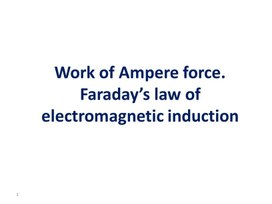3mpere force. Faraday’s law of electromagnetic induction.pptx

## Work of Ampere force. Faraday’s law of electromagnetic induction 1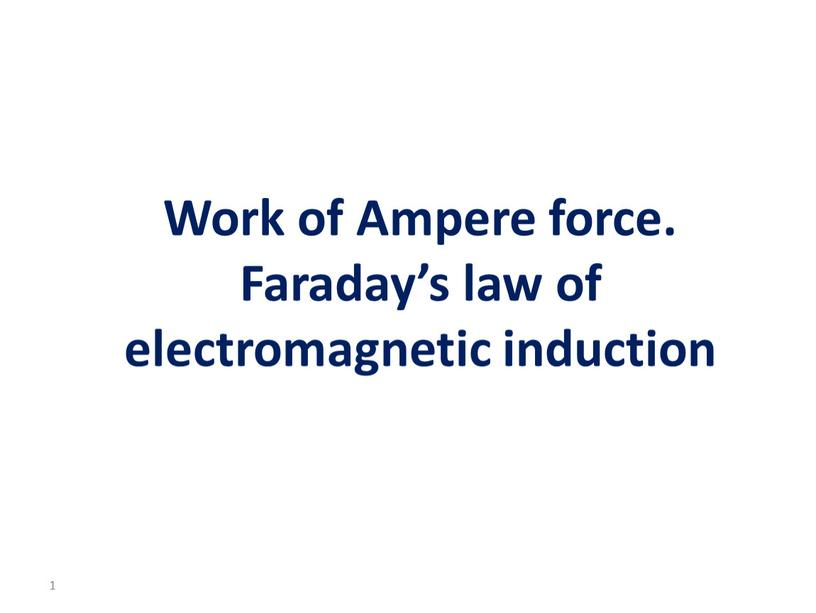Work of Ampere force. Faraday’s law of electromagnetic induction

1

## Learning objective To analyse the operating principle of electromagnetic devices (electromagnetic relay, generator and transformer); 2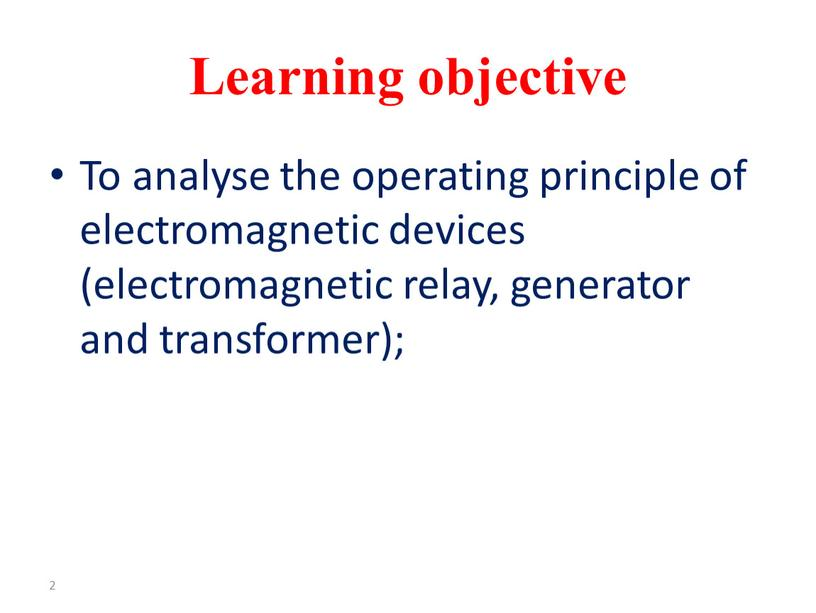Learning objective

To analyse the operating principle of electromagnetic devices (electromagnetic relay, generator and transformer);

2

Discussion: How is the ‘electricity’ made?

The previous demonstrations showed that ‘making’ electricity involves magnetic fields, but what is really going on?

## An electric field E builds up until the force on electrons in the rod due to this electric field (=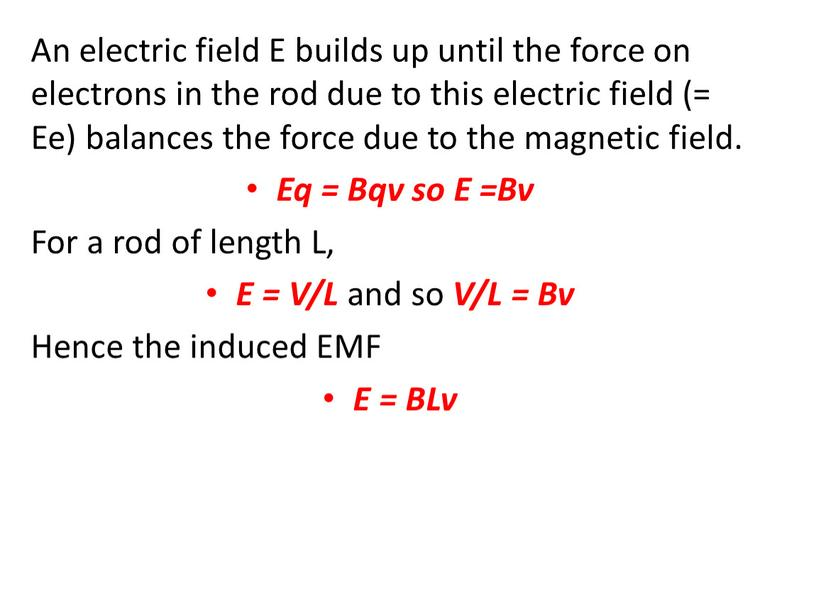An electric field E builds up until the force on electrons in the rod due to this electric field (= Ee) balances the force due to the magnetic field.
Eq = Bqv so E =Bv
For a rod of length L,
E = V/L and so V/L = Bv
Hence the induced EMF
E = BLv

## The EMF will now cause a current to flow in the external resistor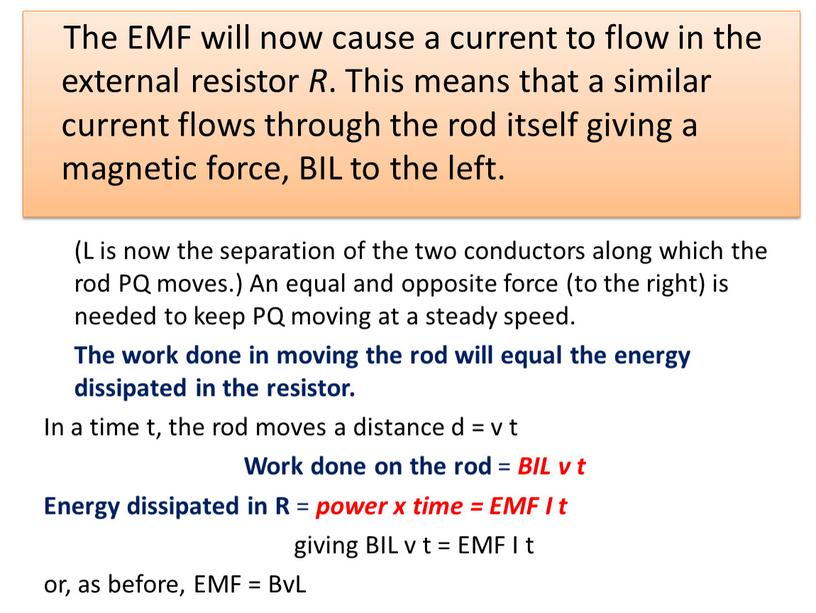The EMF will now cause a current to flow in the external resistor R. This means that a similar current flows through the rod itself giving a magnetic force, BIL to the left.

(L is now the separation of the two conductors along which the rod PQ moves.) An equal and opposite force (to the right) is needed to keep PQ moving at a steady speed.
The work done in moving the rod will equal the energy dissipated in the resistor.
In a time t, the rod moves a distance d = v t
Work done on the rod = BIL v t
Energy dissipated in R = power x time = EMF I t
giving BIL v t = EMF I t
or, as before, EMF = BvL

Induced EMF E = B × area swept out per second = B × A / t
We have already called B the flux density, so it is perhaps not surprising that the quantity B × A can be called the magnetic flux, F.
Thus induced EMF = F / t = rate of change of flux
And more generally E = dF / dt

How can the induced EMF be increased?
moving the wire faster – dA /dt increased –rate of change of flux increased
increasing the field (and hence the flux) –rate of change of flux increased

By increasing the number of turns of wire N in our circuit. By doing this, the flux has not been altered but the flux linkage (N F) will have increased. Hence it is more correct to say that
Induced EMF =  rate of change of flux linkage
E = N × dF/dt

The magnitude of the induced e.m.f. is proportional to the rate of change of magnetic flux linkage.

𝜀= ∆(𝑁Ф) ∆𝑡

where Δ() is the change in the flux linkage in a time Δt.

Lenz's law

The direction of the induced EMF is such that it tends to oppose the motion or change causing it.
To include this idea in our formula, a minus sign has to be introduced, giving;
E = – N ´ dF/dt

## When this bar magnet is thrust into the coil, the strength of the magnetic field increases in the coil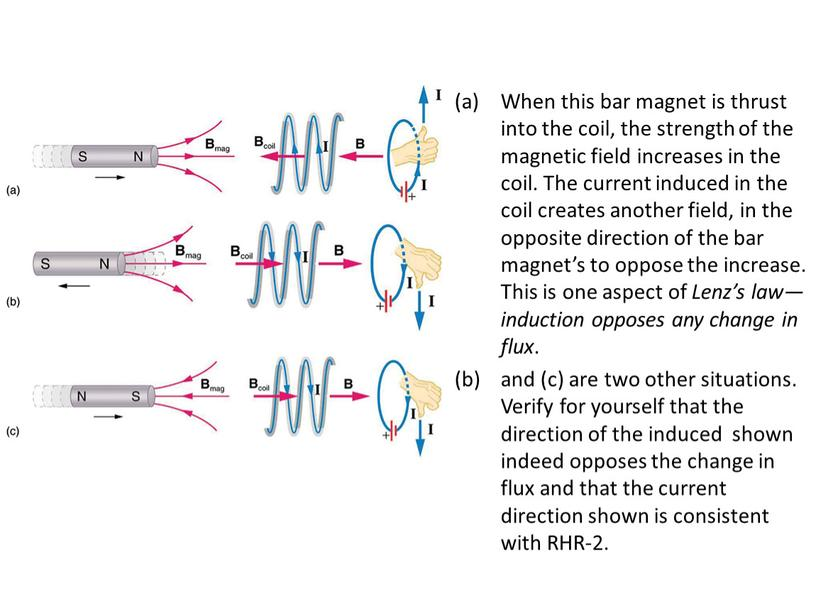When this bar magnet is thrust into the coil, the strength of the magnetic field increases in the coil. The current induced in the coil creates another field, in the opposite direction of the bar magnet’s to oppose the increase. This is one aspect of Lenz’s law—induction opposes any change in flux.
and (c) are two other situations. Verify for yourself that the direction of the induced  shown indeed opposes the change in flux and that the current direction shown is consistent with RHR-2.

Quick Questions Faraday’s Law of Electromagnetic Induction and Lenz’s Law

## 3mpere force. Faraday’s law of electromagnetic inductionReflection

What has been learned
What remained unclear
What is necessary to work on

Скачать файл